• 曲线生成算法的C#gdi+实现（贝塞尔曲线、样条曲线、B样条曲线
• QCharts代码，具有8条通道，可支持8个通道同时显示，也可以单独显示，曲线可以放大缩小移动复原，不能移动放大缩小的曲线都是咸鱼，重构QChartview事件，可暂停继续
• Matlab代码，输入一个Y输出smooth后的Y，适用如下 smoothY = smoothCurve(Y);STM32F1
• 基于python实现串口通讯，从串口读取数据后进行动态曲线绘制，然后将数据保存到文件中，曲线绘制可实现暂停
• dubins详细代码，matlab版本，适用于航迹规划、路径规划，求满足约束的两点之间最短路径
• LineWaves-2.0，Curvy，Vectrosity 5.4.2,三种强大的画线工具，仅供学习使用。
• 用余弦相似度与距离差比较曲线相似度，提出位移比较法对曲线初始位置不一致有较好处理效果，纯C代码未调第三方库，所有原理与代码均有注解，适合数据处理与图像处理用
• 内包含STM32电机S/Spta算法控制源码例程（PWM/定时器基准，已亲测应用），附带加减速效果曲线加速器和步进伺服电机曲线计算参数表，以及核心算法说明。
• 相关文章请看：https://blog.csdn.net/bwangk/article/details/82082133
• 使用python进行线性拟合和曲线拟合，包括多项式函数和幂指数函数等曲线拟合，可以导入excel数据，并进行相关系数、可决系数、均方误差的求取，以及进行曲线可视化。
• 贝塞尔曲线c++实现。鼠标右键创建端点，左键移动端点，中键回溯。可以用vs或QT creator打开。这是完整的项目文件夹，保证编译通过。新版更新了优化了一下代码，解决了右键拖动经常拖不动的问题。
• 用于yolo计算mAP和PR曲线使用，里面有4个python文件，分为python2和python3版本
• 对多个点组成的数组进行插值拟合，在折点处得到光滑的曲线
• 点到点轨迹规划的S曲线，已知起始位置、终止位置、最大速度、最大加速度、总的运动时间、这5个参数，自动计算出运动规划曲线（若输入的参数不合适，代码可以自行计算出合适参数）
• C#图谱曲线平滑滤波算法，几个平滑直接用。其实平滑算法是触类旁通的。
• 这是一个用Qt实现的B样条曲线的演示程序，源代码的下载链接在我的博客或者我的其他下载资源中可以找到：
• 随机生成一组数据，QT用Qpainter绘制实时曲线图最简单的折线图
• ## 最小二乘法曲线拟合以及Matlab实现

万次阅读 多人点赞 2017-12-28 17:31:23
最小二乘法曲线拟合以及Matlab实现 在实际工程中，我们常会遇到这种问题：已知一组点的横纵坐标，需要绘制出一条尽可能逼近这些点的曲线（或直线），以进行进一步进行加工或者分析两个变量之间的相互关系。而获取这...
最小二乘法曲线拟合以及Matlab实现
在实际工程中，我们常会遇到这种问题：已知一组点的横纵坐标，需要绘制出一条尽可能逼近这些点的曲线（或直线），以进行进一步进行加工或者分析两个变量之间的相互关系。而获取这个曲线方程的过程就是曲线拟合。
目录
最小二乘法直线拟合原理曲线拟合Matlab实现代码

最小二乘法直线线拟合原理
首先，我们从曲线拟合的最简单情况——直线拟合来引入问题。如果待拟合点集近似排列在一条直线上时，我们可以设直线

y

=

a

x

+

b

y=ax+b

为其拟合方程，系数

A

=

[

a

,

b

]

A=[a,b]

为待求解项，已知：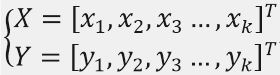。
用矩阵形式表达为：

Y

=

X

0

A

Y=X_{0}A

，其中：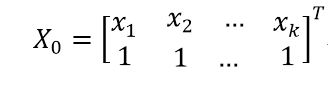要求解A，可在方程两边同时左乘

X

0

X_{0}

的逆矩阵，如果它是一个方阵且非奇异的话。
但是，一般情况下

X

0

X_{0}

连方阵都不是，所以我们在此需要用

X

0

X_{0}

构造一个方阵，即方程两边同时左乘

X

0

X_{0}

的转置矩阵，得到方程:

X

0

T

Y

=

X

0

T

X

0

A

X_{0}^{T}Y=X_{0}^{T}X_{0}A

。
此时，方程的系数矩阵

X

0

T

X

0

X_{0}^{T}X_{0}

为方阵，所以两边同时左乘新系数矩阵

X

0

T

X

0

X_{0}^{T}X_{0}

的逆矩阵，便可求得系数向量A ，即：

(

X

0

T

X

0

)

−

1

X

0

T

Y

=

A

(X_{0}^{T}X_{0})^{-1}X_{0}^{T}Y=A

。
方程

A

=

(

X

0

T

X

0

)

−

1

X

0

T

Y

A=(X_{0}^{T}X_{0})^{-1}X_{0}^{T}Y

右边各部分均已知，所以可直接求解得到拟合直线的方程系数向量A。
曲线线拟合
当样本点的分布不为直线时，我们可用多项式曲线拟合，即拟合曲线方程为n阶多项式

y

=

∑

i

=

0

n

a

i

x

i

=

a

n

x

n

+

a

n

−

1

x

n

−

1

+

.

.

.

+

a

1

x

+

a

0

y=\sum_{i=0}^{n}a_ix^i=a_nx^n+a_{n-1}x^{n-1}+...+a_1x+a_0

。
用矩阵形式表示为：

Y

=

X

0

A

Y=X_0A

，其中：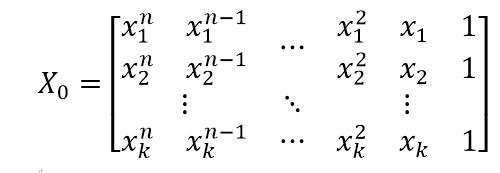待求解项为系数向量

A

=

[

a

n

,

a

n

−

1

,

.

.

.

,

a

2

,

a

1

,

a

0

]

T

A=[a_n,a_{n-1},...,a_2,a_1,a_0]^T

。
曲线拟合方程

Y

=

X

0

A

Y=X_0A

的求解方法与上面直线的求解方法一样，也是在方程

Y

=

X

0

A

Y=X_0A

两边同左乘

X

0

X_0

的转置矩阵得到:

X

0

T

Y

=

X

0

T

X

0

A

X_{0}^{T}Y=X_{0}^{T}X_{0}A

，
再同时在新方程两边同时左乘

X

0

T

X

0

X_{0}^{T}X_{0}

的逆矩阵，得到：

(

X

0

T

X

0

)

−

1

X

0

T

Y

=

A

(X_{0}^{T}X_{0})^{-1}X_{0}^{T}Y=A

上式左边各部分均已知，所以可直接求解得拟合曲线方程的系数向量A。
Matlab实现代码
%by hanlestudy@163.com
clear
clc
x=[2,4,5,6,6.8,7.5,9,12,13.3,15];
y=[-10,-6.9,-4.2,-2,0,2.1,3,5.2,6.4,4.5];
[~,k]=size(x);
for n=1:9
X0=zeros(n+1,k);
for k0=1:k           %构造矩阵X0
for n0=1:n+1
X0(n0,k0)=x(k0)^(n+1-n0);
end
end
X=X0';
ANSS=(X'*X)\X'*y';
end
x0=0:0.01:17;
y0=ANSS(1)*x0.^n    ;%根据求得的系数初始化并构造多项式方程
for num=2:1:n+1
y0=y0+ANSS(num)*x0.^(n+1-num);
end
subplot(3,3,n)
plot(x,y,'*')
hold on
plot(x0,y0)
end
suptitle('不同次数方程曲线拟合结果，从1到9阶')

运行结果
拟合曲线结果：
可以看出看来，当多项式的阶数过小是，曲线并不能很好地反映出样本点的分布情况；但阶数过高时，会出现过拟合的情况。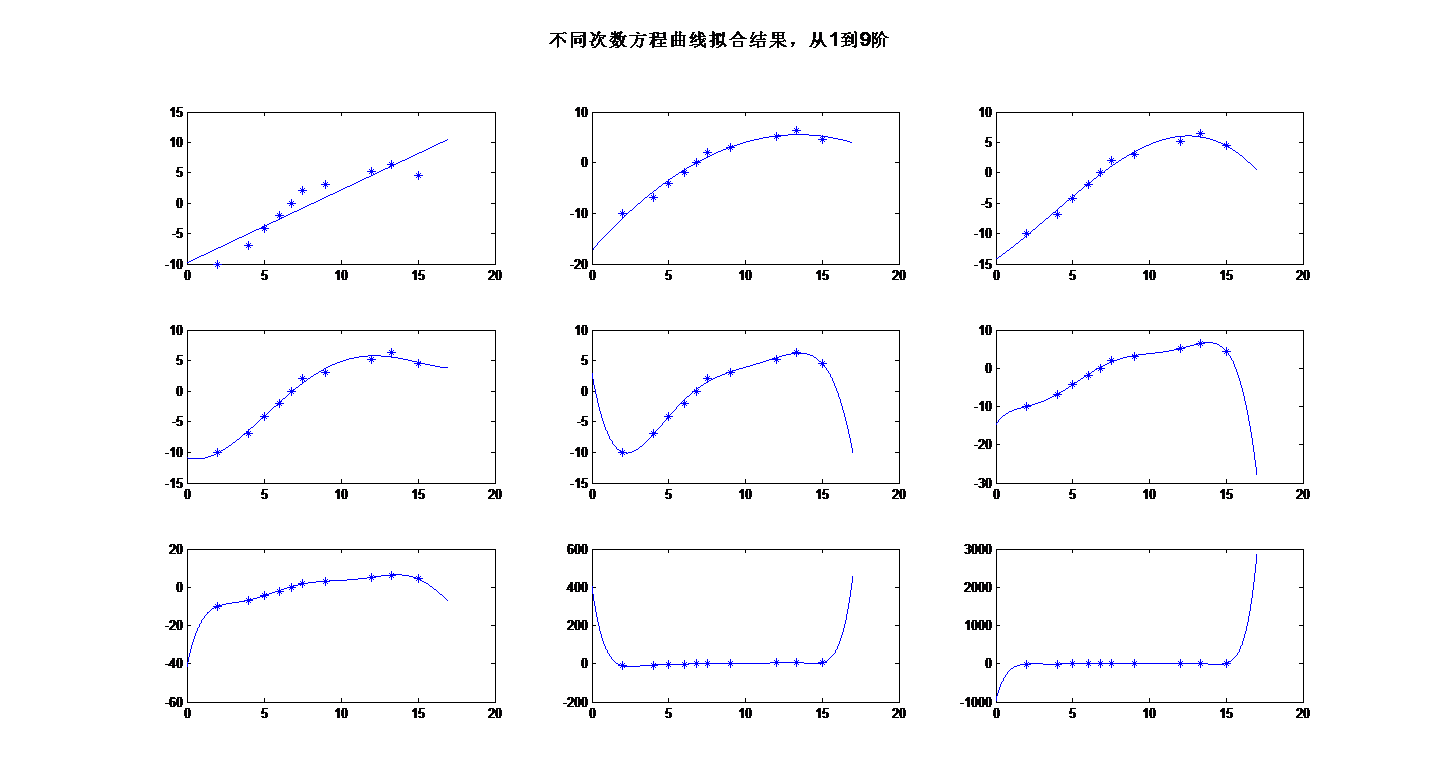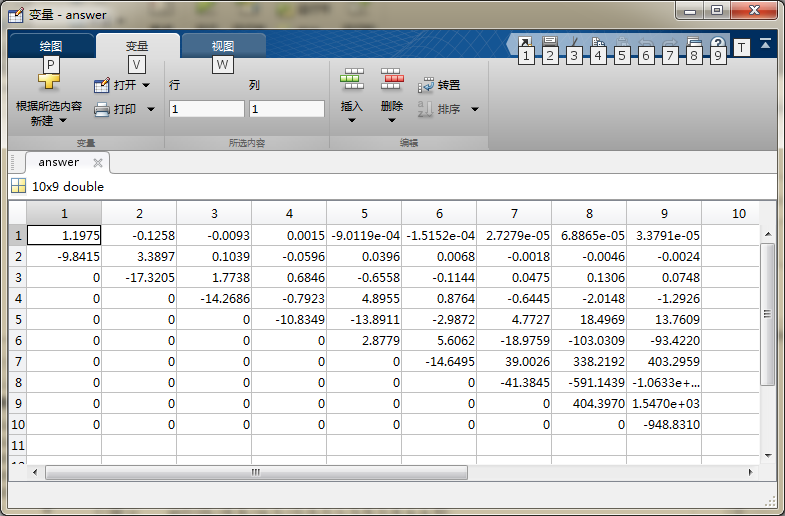Matlab自带函数——polyfit
在matlab中，也有现成的曲线拟合函数polyfit，其也是基于最小二乘原理实现的，具体用法为：ans=polyfit(x,y,n). 其中x,y为待拟合点的坐标向量，n为多项式的阶数。
下面代码是用polyfit函数来做曲线拟合：
clear
clc
x=[2,4,5,6,6.8,7.5,9,12,13.3,15];
[~,k]=size(x);
y=[-10,-6.9,-4.2,-2,0,2.1,3,5.2,6.4,4.5];
for n=1:9
ANSS=polyfit(x,y,n);  %用polyfit拟合曲线
end
x0=0:0.01:17;
y0=ANSS(1)*x0.^n    ; %根据求得的系数初始化并构造多项式方程
for num=2:1:n+1
y0=y0+ANSS(num)*x0.^(n+1-num);
end
subplot(3,3,n)
plot(x,y,'*')
hold on
plot(x0,y0)
end
suptitle('不同次数方程曲线拟合结果，从1到9阶')

运行结果：
用polyfit拟合的结果与第一份代码运行的结果基本一样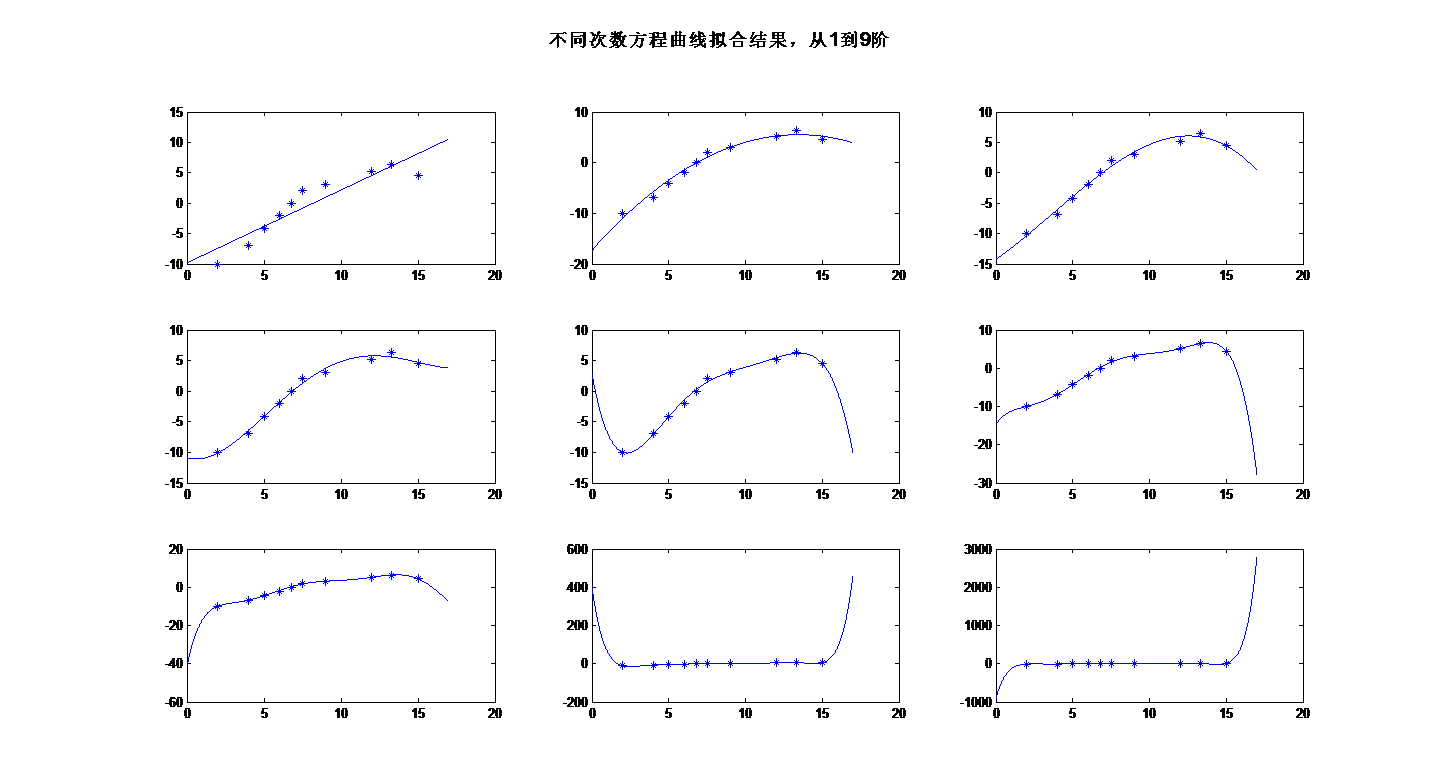申明
本文为本人原创，转载请注明出处！
展开全文最小二乘法 matlab
• 使用的是Qt4开发，经过实际的测试完全可以用，使用Qt5，只需简单的修改头文件也可以用。 在本人的博客中也有详细的程序解读，和开发的重要注意。
• ## ROC曲线的绘制

千次阅读 2019-10-15 21:21:22
ROC（Receiver Operating Characteristic）曲线全称是“受试者工作特征”，通常用来衡量一个二分类学习器的好坏。如果一个学习器的ROC曲线能将另一个学习器的ROC曲线完全包住，则说明该学习器的性能优于另一个学习器。
在绘制ROC曲线的时候，首先明白ROC曲线的横纵坐标分别代表的是什么。
下面介绍下混淆矩阵。在评价二分类器会经常用到的表格。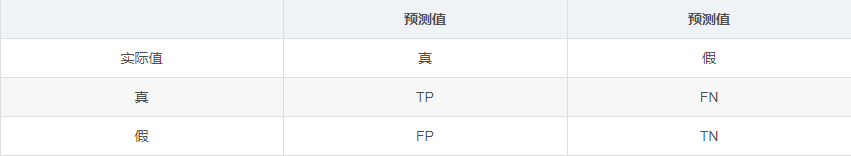纵坐标：TPR=TP/(TP+FN)
横坐标：FPR=TP/(FP+TN)
那么上面的指标已经介绍完了，如何根据这些指标去绘制合适的ROC曲线呢：
实际上对于许多学习器在判定二分类问题时是预测出一个对于真值的范围在[0.0, 1.0]之间的概率值，而判定是否为真值则看该概率值是否大于设定的阈值（Threshold）。例如如果阈值设定为0.5则所有概率值大于0.5的均为正例，其余为反例。因此对于不同的阈值我们可以得到一系列相应的FPR和TPR，从而绘制出ROC曲线。
y_true = [0, 1, 0, 1]，真实值
y_score = [0.1, 0.35, 0.4, 0.8]， 预测概率值
分别取4组判定正例用的阈值：[0.1, 0.35, 0.4, 0.8]，可得相应4组FPR,TPR:
FPR: [1, 0.5, 0.5, 0]  TPR: [1, 1, 0.5, 0.5]
则可以根据这四个点绘制出对应的ROC曲线了。
ROC曲线所覆盖的面积称为AUC（Area Under Curve），可以更直观的判断学习器的性能，AUC越大则性能越好。对于该例的AUC值为0.75。
展开全文• C#实现显示实时数据，并形成曲线，保存数据，完整的项目代码
• 用于学习贝塞尔曲线原理，观察贝塞尔曲线。并初步了解曲线绘制方法。
• 汇总的Android下常见曲线图，附带源码，有些没有验证过
• 在MATLAB中创建GUI，能够自由拖动点以调试曲线，且能自由移动坐标轴，放大图像，保存曲线中的点
• ## android 实时曲线图

热门讨论 2014-12-12 12:56:27
利用achartengine实现的实时曲线图，其中有两种实现方式（含四种表现方式），使用时请先将android-support-v7-appcompat导入，然后导入RealTimeChart，导入完成后，修改Library引用，方法如下：右击RealTimeChart...
• nurbs曲线绘制程序，matlan程序代码，内含详细注释说明nurbs
• 本程序基于蒙特卡洛思想生成电动汽车充电负荷曲线，利用第十一届电工杯所提供的数据（充电开始时间，充电电量，充电功率）得到一万台电动汽车充电负荷曲线。蒙特卡洛只是解决问题的一种思想，本程序可为其他利用...
• 绘制（平滑与折线）曲线。 数据直观，界面漂亮。Qt界面...